Next: Motion with constant acceleration Up: Motion in 1 dimension Previous: Acceleration

## Motion with constant velocity

The simplest type of motion (excluding the trivial case in which the body under investigation remains at rest) consists of motion with constant velocity. This type of motion occurs in everyday life whenever an object slides over a horizontal, low friction surface: e.g., a puck sliding across a hockey rink.

Fig. 6 shows the graph of displacement versus time for a body moving with constant velocity. It can be seen that the graph consists of a straight-line. This line can be represented algebraically as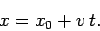(18)

Here,is the displacement at time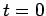: this quantity can be determined from the graph as the intercept of the straight-line with the-axis. Likewise,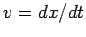is the constant velocity of the body: this quantity can be determined from the graph as the gradient of the straight-line (i.e., the ratio, as shown). Note that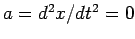, as expected.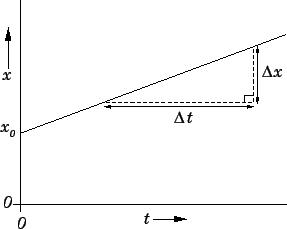Fig. 7 shows a displacement versus time graph for a slightly more complicated case of motion with constant velocity. The body in question moves to the right (sinceis clearly increasing with) with a constant velocity (since the graph is a straight-line) between timesand. The body then moves to the right (sinceis still increasing in time) with a somewhat larger constant velocity (since the graph is again a straight line, but possesses a larger gradient than before) between timesand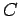. The body remains at rest (since the graph is horizontal) between timesand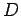. Finally, the body moves to the left (sinceis decreasing with) with a constant velocity (since the graph is a straight-line) between timesand.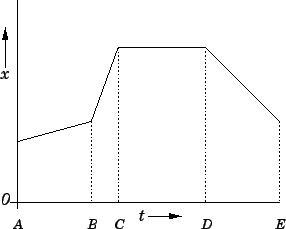Next: Motion with constant acceleration Up: Motion in 1 dimension Previous: Acceleration
Richard Fitzpatrick 2006-02-02